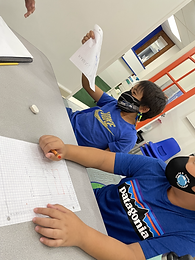## Ms. Kayla

### Target 1​

###### Lesson Type:

Review

Geometry

:

Shape

Classify 2D and 3D figures based on the basic properties of shapes.

###### 2:

Identify quadrilaterals by their defining attributes (number of sides, number of vertices, vertices angle measurements, line types, and side lengths).

###### 3:

Identify parallel and perpendicular lines.

###### 4:

Define each type of quadrilateral (square, rectangle, parallelogram, rhombus, and trapezoid).

5th

###### Vocabulary:

Quadrilateral, Attribute, Difference, Parallel, Perpendicular, Congruent

Activities:

1. Students reviewed the types of quadrilaterals and their attributes, how they are different from one another.

- Students looked at the similarities and differences between each quadrilateral.

- Students identified the types of lines (parallel, perpendicular), angles, and congruency.### Home Exploration

###### Guiding Questions:## Absent Students:

### Target 2

:

###### 1:

Understand that geometric figures can be drawn on the coordinate plane.

###### 2:

Draw quadrilaterals on the coordinate plane.

5th

###### Vocabulary:

Activities:

1. Students plotted points on a coordinate grid to create a quadrilateral. Each point was identified with a coordinate pair.

2. Students then listed their coordinate pairs for others to plot on their coordinate plane/grid. Students had to identify which quadrilateral could be drawn from the listed coordinate pairs.### Home Exploration

###### Guiding Questions:### Target 3

:

###### Vocabulary:

Activities:### Home Exploration